# Routing with GRASS GIS: Catchment Area Calculation

I got my hands on pgRouting in the last post and I’m about to do the same with GRASS GIS in this one.

GRASS GIS stores the topology for the native vector format by default, which makes it easy to use for the network analysis. All the commands associated with the network analysis can be found in the `v.net` family. The ones I’m going to discuss in this post are `v.net` itself, `v.net.path`, `.v.net.alloc` and `v.net.iso`, respectively.

## Data

I’m going to use the roads data from the previous post together with some random points used as catchment areas centers.

```# create the new GRASS GIS location
grass -text -c ./osm/czech

# import the roads
v.in.ogr input="PG:host=localhost dbname=pgrouting" layer=cze.roads output=roads -eo  --overwrite

# import the random points
v.in.ogr input="PG:host=localhost dbname=pgrouting" layer=temp.points output=points -eo --overwrite
```

I got six different points and the pretty dense road network. Note none of the points is connected to the existing network.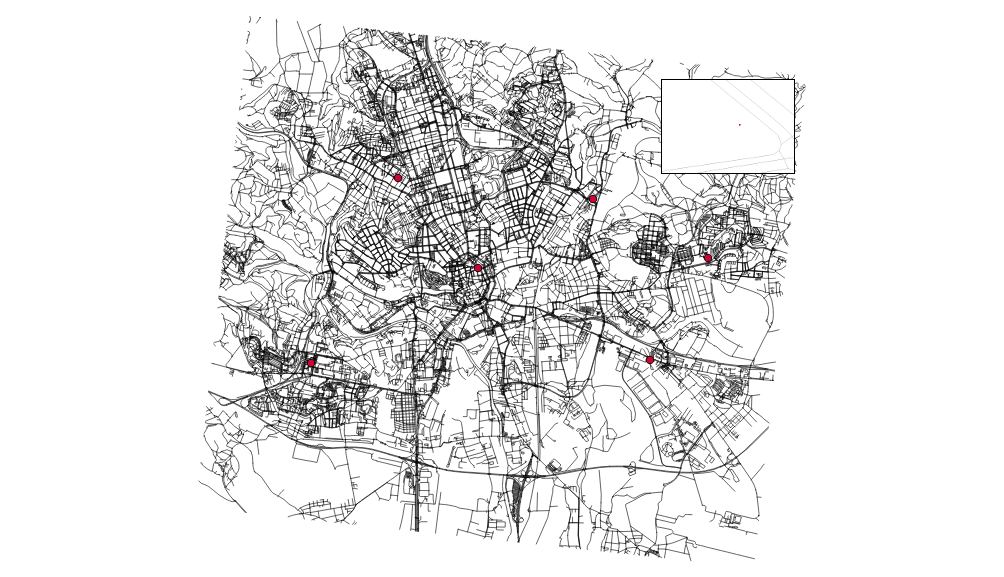You have to have routable network to do the actual routing (the worst sentence ever written). To do so, let’s:

• connect the random points to the network
• add nodes to ends and intersections of the roads

Note I’m using the 500m as the max distance in which to connect the points to the network.

```v.net input=roads points=points operation=connect threshold=500 output=network
v.net input=network output=network_noded operation=nodes
```

## Finding the shortest path between two points

Once the network is routable, it is easy to find the shortest path between points number 1 and 4 and store it in the new map.

```echo "1 1 4" | v.net.path input=network_noded output=path_1_4
```

The algorithm doesn’t take bridges, tunnels and oneways into account, it’s capable of doing so though.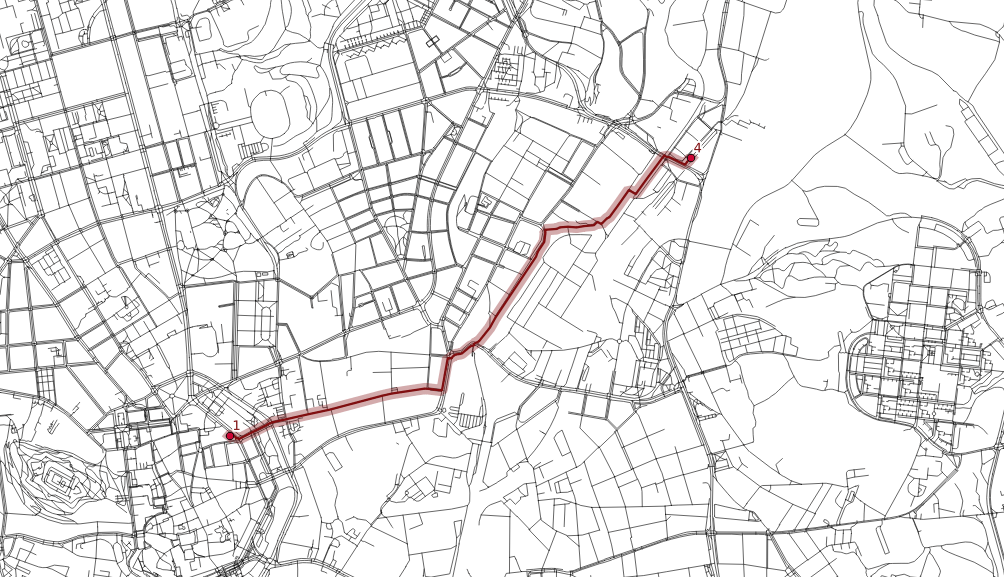## Distributing the subnets for nearest centers

```v.net.alloc input=network_noded output=network_alloc center_cats=1-6 node_layer=2
```

`v.net.alloc` module takes the given centers and distributes the network so each of its parts belongs to exactly one center - the nearest one (speaking the distance, time units, …).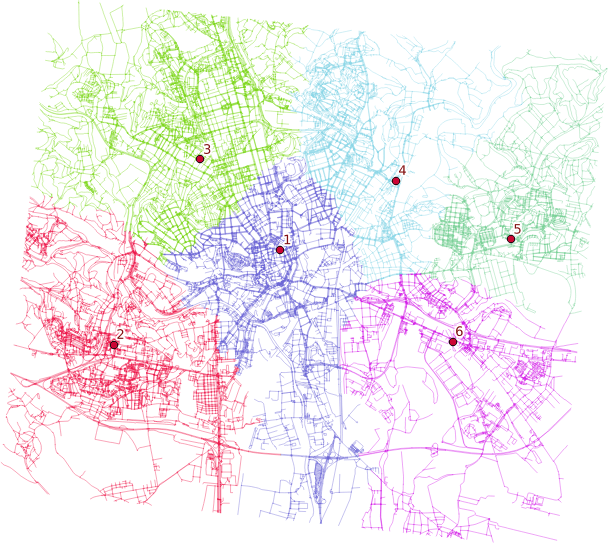## Creating catchment areas

```v.net.iso input=network_noded output=network_iso center_cats=1-6 costs=1000,3000,5000
```

`v.net.iso` splits net by cost isolines. Again, the costs might be specified as lengths, time units, ….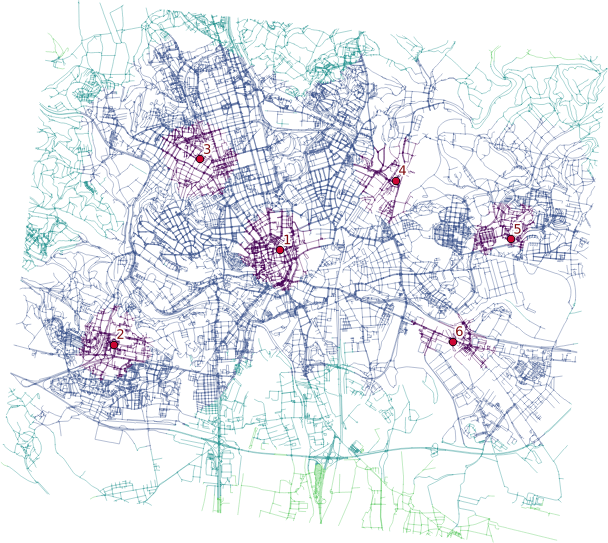Two different ways lead to the actual catchment area creation. First, you extract nodes from the roads with their values, turn them into the raster grid and either extract contours or polygonize the raster. I find the last step suboptimal and would love to find another way of polygonizing the results.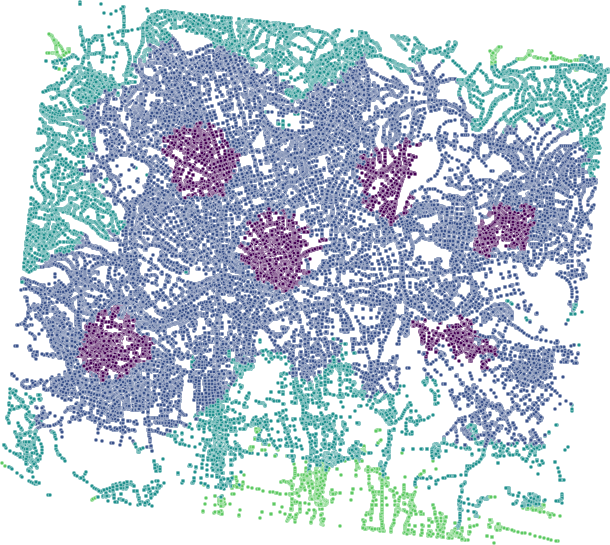Note when extracting contours the interval has to be set to the reasonable number depending on the nodes values.

## Remarks

• Once you grasp the basics, GRASS GIS is real fun. Grasping the basics is pretty tough though.
• Pedestrians usually don’t follow the road network.
• Bridges and tunnels might be an issue.
• Personally, I find GRASS GIS easier to use for the network analysis compared to pgRouting.